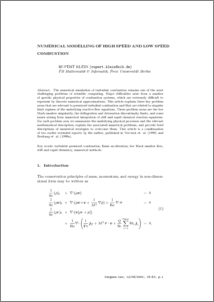Repository: Freie Universität Berlin, Math Department

# Numerical Methods for High and Low Speed Combustion Simulations

Klein, R. (2002) Numerical Methods for High and Low Speed Combustion Simulations. Numerical Methods for High and Low Speed Combustion Simulations. PDE's in models of superfluidity, superconductivity and reactive flows, Nato S (569). pp. 491-506.Preview

723kB

## Abstract

The numerical simulation of turbulent combustion remains one of the most challenging problems of scientific computing. Major difficulties arise from a number of specific physical properties of combustion systems, which are extremely difficult to represent by discrete numerical approximations. This article explains three key problem areas that are relevant to premixed turbulent combustion and that are related to singular limit regimes of the underlying reactive °ow equations. These problem areas are the low Mach number singularity, the deflagration and detonation discontinuity limits, and some issues arising from numerical integration of stiff and rapid chemical reaction equations. For each problem area we summarize the underlying physical processes and the relevant mathematical description, explain the associated numerical problems, and provide brief descriptions of numerical strategies to overcome them. This article is a condensation of two earlier extended reports by the author, published in Vervisch et al. (1999) and Breitung et al. (1999a).

Item Type: Article Mathematical and Computer Sciences > Mathematics > Applied Mathematics Department of Mathematics and Computer Science > Institute of Mathematics > Geophysical Fluid Dynamics Group 683 Ulrike Eickers 31 Jul 2009 14:49 03 Mar 2017 14:40

Repository Staff Only: item control page